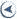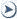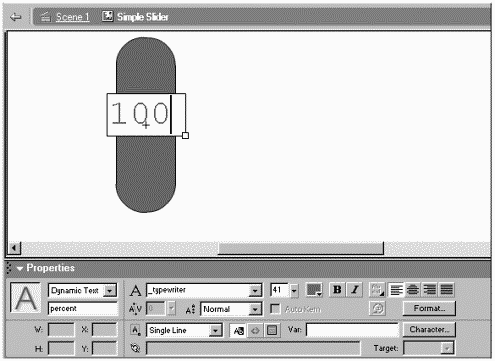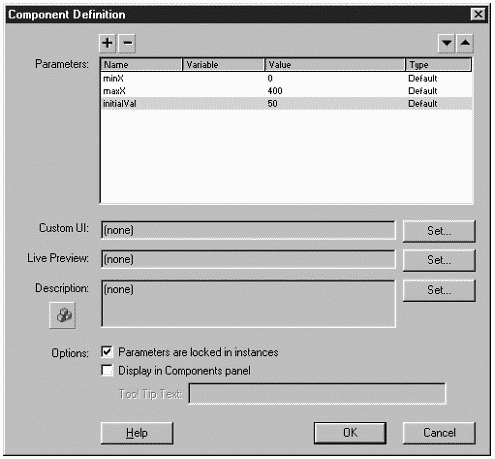# Workshop Chapter 3. Creating a Horizontal SliderCONTENTS•  Creating a Quick-and-Dirty Slider
•  Converting the Slider into a Component
•  Summary

This workshop explores a popular user interface control: the slider. Many users prefer to specify a number by using the seemingly continuous scale offered by a slider rather than by typing into a field. Some tactile learners may respond better to the sense of touch provided by a slider. Another benefit of using sliders is that you can adjust them without taking your hand off the mouse.

This workshop has two parts. First we make a perfectly acceptable plain slider, and then we convert it into a more useful component.

### Creating a Quick-and-Dirty Slider

1. Create a shape that will serve as the slider the users are supposed to grab. Convert it to a Movie Clip symbol called "Simple Slider." Perhaps you'll want to use a vertical rectangle with rounded corners.

2. Making the slider movable is easy enough but we just need it to move horizontally, and only while users are dragging it. To move the slider only while users are dragging it, we'll set a homemade variable (active) to true when they press and then false when they let go. Then, for every mouseMove event, we'll only move the clip if the active variable is true. First name the instance of clip to slider, and then put the following code in the frame of the main timeline:

`slider.onPress=function(){   this.active=true;  }  slider.onRelease=function(){   this.active=false;  }  slider.onReleaseOutside=function(){   this.active=false;  }`

Notice that there are two ways to "unclick": onRelease and onReleaseOutside. That is, if users press the mouse on the slider and keep holding their mouse until it's outside the Flash window, only onReleaseOutside will capture that event.

3. Now you can add the code for an onMouseMove callback function (anywhere in this same frame):

`slider.onMouseMove=function(){   if(this.active){      this._x=_xmouse;       updateAfterEvent();    }  }`

Now, any time the mouse moves, this code sets the clip's _x (this._x) to the mouse's _x (provided that the variable active is true).

You can test the movie now and see we're getting pretty close. It would be nice to set some limits for the high and low points, and to see a percentage displayed as it slides. The limits are pretty easy. We'll start by setting arbitrary (and hard-wired) values, and then improve on it later.

4. First determine the minimum and maximum x positions for the slider instance. You can draw a few vertical lines and then snap the slider to those lines taking note as to what is displayed in Info panel. (Remember to use the center point option when gathering this information instead of the top-left option.) In my case, I decided upon a minimum of 50 and maximum of 250 (yes, to make it easier later) but you can use any numbers you want. Because I know I'm going to need access to the minimum and maximum values later, they should be stored in a variable. Here are two lines of code that can precede everything else you've written:

`slider.minX=50;  slider.maxX=250;`

I decided to store my two variables inside the slider instance itself.

5. Now we can adjust the onMouseMove callback so that the slider never goes past the limits. There are often two ways to approach this issue: to prevent the slider from ever going past the limits, or to let it go past the ends but fix it before anyone sees anything. I'm going to opt for the second choice that is, if the code places the slider past the end points, I'll fix it. You can see the code I came up with is pretty wordy but easy to follow. Use this replacement for the onMouseMove callback:

`slider.onMouseMove=function(){   if(this.active){     this._x=_xmouse;      if(this._x>this.maxX){       this._x=this.maxX;      }      if(this._x<this.minX){       this._x=this.minX;      }      updateAfterEvent();    }  }`

Those two if statements just say, "If it's greater than the max, set it to the max; if it's less than the minimum, set it to the minimum." I find this "fix it when there's a problem" approach is usually easier than trying to avoid any possibility of users breaching the limits. Users won't see anything refresh onscreen until this function is complete, so it doesn't matter.

6. Now we can display the percentage. Double-click the slider instance to edit the contents of the Simple Slider symbol. Create a Dynamic Text field and give it an instance name percent. Make sure the margins are wide enough to display 100 (actually, just type 100 into the field). You can position it next to or on top of the slider shape. (See Figure W3.1.) Now go back up to the main timeline so that we can edit the scripts.

##### Figure W3.1. A Dynamic Text field inside the Simple Slider symbol will display a percentage.The formula for percentage is simply "current position divided by the total distance." Actually, that formula will yield percentages from 0 to 1. For example, if the current position is the same as the total, you'll get 1. Multiplying by 100 gives us the right formula: current/total*100. You can try a few "what-if" numbers to confirm this formula. Now, translating that formula to our application is not very difficult at all. First, let's try a few easy examples to deduce a formula. My minimum was 50 and my maximum was 250. What would the percentage be if the current position was 150? Sketch it out and I'll bet you know it's 50 percent. That's because the total difference is 200 (250-50) and the current position (150) was really an "elapsed" distance of 100 (because it started at 50). So the current position is 150-50. By replacing those numbers with dynamic expressions based on our variables, the percentage is returned from the expression:

`(_x-minX) / (maxX-minX) *100`

Replace the variables and replace _x with 150 and you have:

`(150-50) / (250-50) *100`

It helps to make up nice numbers when testing such formulas.

7. Now we can add one line of code to complete our onMouseMove callback:

` 1 slider.onMouseMove=function(){  2   if(this.active){  3     this._x=_xmouse;   4     if(this._x>this.maxX){  5       this._x=this.maxX;   6     }   7     if(this._x<this.minX){  8       this._x=this.minX;   9     }  10     this.percent.text=(this._x-this.minX)/(this.maxX-this.minX) *100;  11     updateAfterEvent();  12   }  13 }`

Notice line 10, which set the text property of the percent instance. If you test it now, you'll see the percent is displaying decimal values, which is really more than we need. To display only the integer portion of the value found, change line 10 to:

`this.percent.text=Math.floor((this._x-this.minX)/(this.maxX-this.minX) *100);`

Go ahead and test the movie to see if you can find anything you think we should fix. There are just a couple minor touches that I think are worth fixing before we convert it to a component. First, I don't like the way the slider always snaps a tiny bit unless I grab it right by the center. The problem is that the slider is being placed at the exact _xmouse location. Also, the slider doesn't display the correct percentage until I start sliding it. Finally, it would be nice if this slider did something such as control the _alpha level of another clip or the volume of a sound.

Although fixing the snap issue is pretty easy, it's important to fully understand the problem and exactly what you want it to do differently. The problem is that the slider centers itself to where the mouse is. Say you click on the right side to start dragging. We'd like (as the slider gets dragged) for it to position itself to where the mouse is minus a little bit. When you click on the left, we want the slider to be where the mouse is plus a little. Exactly how much extra is added or subtracted depends on how far off center you click. So the fix is two-part: First, use a variable to save how far off center they click. Then, use that variable's value when moving the slider.

8. To fix the snap issue, change the onPress callback by adding one line of code so that it reads as follows:

`slider.onPress=function(){   this.offSet=this._x-_xmouse;    this.active=true;  }`

The second line simply stores (into the variable offSet) the difference between the slider's _x and the _xmouse.

9. Now, adjust the third line inside the onMouseMove callback (from earlier) so that when you set the _x of the slider you set it to the _xmouse plus offSet:

`this._x=_xmouse+this.offSet;`
10. To make the slider display the correct percentage at the very beginning, place the following code below all the other code:

`slider.percent.text=Math.floor((slider._x-slider.minX)/(slider.maxX-slider.minX)*100);`

Basically, this is the same code from inside the onMouseMove callback (line 10 shown previously), but I changed all the this's to address slider. I realize it's sort of ugly and long, but we'll fix this later (when converting to a component).

11. Finally, to make this slider do something, we'll just invoke a function and send the current percent as a parameter. We can define this function in the same frame with all the rest of the scripts:

`function doit(howMuch){   slider._alpha=howMuch;  }`

This function sets the _alpha of the slider to whichever value is received as a parameter. You can change the code inside the function to affect any clip you want, in any way. For example, you might have another instance called dial that you want to rotate. You could change the code to:

`dial._rotation=(howMuch/100)*360;`
12. To trigger the doit() function (and send the percentage), we'll add an extra line to the onMouseMove callback. Because we need to both display percentage and send it as a parameter, I decided to add a local variable p. Notice that where I used to set the text of percent, I now do three things (lines 10 12): calculate the percentage and save it in a variable p, set the text property, and trigger the doit() function:

` 1 slider.onMouseMove=function(){  2   if(this.active){  3     this._x=_xmouse+this.offSet;   4     if(this._x>this.maxX){  4       this._x=this.maxX;   5     }   6     if(this._x<this.minX){  7       this._x=this.minX;   8     }   9     var p=Math.floor((this._x-this.minX)/(this.maxX-this.minX)*100);  10     this.percent.text=p;  11     doit(p);  12     updateAfterEvent();  13   }  14 }`

This is a pretty solid slider now. You could probably spend more time refining it even further, but (for better or worse) other issues will arise when converting it to a component, so it makes sense to move on (and deal with the other issues if and when they arise). Sometimes just knowing which issues remain is all that's necessary; they sometimes fix themselves or become moot. Anyway, we're moving on to make this a component.

### Converting the Slider into a Component

We're about to make our slider slightly better. The limiting issues with the slider we built in the first part of this workshop include the following:

• All the code is in the main timeline. The problem is that we can't drag additional instances of this clip from the Library without copying and pasting the code and naming the slider instance. If there were a bug in the code, it could get duplicated and would have to be fixed for each case.

• The minX and maxX variables are hard-wired. Our component will allow each instance its own minX and maxX values, plus an additional variable for the starting percentage.

Here are the steps for making our pretty good slider into a very good component that addresses the preceding issues:

1. The first step is to encapsulate all the code and graphics into a single symbol. Select all the code (in the first frame) and cut it. Then, double-click the slider instance to edit the master symbol. Paste the code into the first frame inside the Simple Slider symbol. Now we just have to adjust it a bit.

2. Because we are now inside the slider instance, you can change the code to say this anywhere it currently says slider. There's actually one other replacement that you may notice in the finished code that goes inside the clip:

` 1 this.minX=0;   2 this.maxX=330;   3   4 this.onPress=function(){  5   this.offSet=this._x-_parent._xmouse;   6   this.active=true;   7 }   8 this.onRelease=function(){  9   this.active=false;  10 }  11 this.onReleaseOutside=function(){ 12   this.active=false;  13 }  14  15 this.onMouseMove=function(){ 16   if(this.active){ 17     this._x=_parent._xmouse+this.offSet;  18     if(this._x>this.maxX){ 19       this._x=this.maxX;  20     }  21     if(this._x<this.minX){ 22       this._x=this.minX;  23     }  24     var p=Math.floor((this._x-this.minX)/(this.maxX-this.minX)*100);  25     this.percent.text=p;  26     doit(p);  27     updateAfterEvent();  28   }  29 }  30 function doit(howMuch){ 31   this._alpha=howMuch;  32 }  33 this.percent.text=Math.floor((this._x-this.minX)/(this.maxX-this.minX)*100);`

Notice in lines 5 and 17 that _xmouse changed to _parent._xmouse because the hierarchy has changed. This code is much better even if we don't make a component because now we can drag as many instances of the Simple Slider onto the Stage and each will perform independently. You don't even need to give them instance names!

3. Now we can convert to a component. First, we need to decide which variables are going to be set by the using author. Actually, we can add more later, but we already know that minX and maxX should be specified by the using author. In addition, the initial percentage should be set-able by the using author. Let's call that variable initialVal.

4. To make this a component (and give the using author access to the three variables minX, maxX, and initialVal), open the Library, select the Simple Slider symbol, and then select Component Definition from the options menu. Click the Plus button three times, and then change the three names ("varName") minX, maxX, and initialVal. In the Value column, change "defaultValue" to 0 for minX, 400 for maxX, and 50 for initialVal. These are just in case the using author never populates these values. You can see how the Component Definition dialog box looks in Figure W3.2. Click OK when you're done.

##### Figure W3.2. Our slider becomes a component after we configure the Component Definition dialog box.5. Now that the using author will set minX and maxX, we don't need the first two lines of code inside the symbol. Go ahead and comment out (with //) the first two lines of code inside our component where minX and maxX are assigned. By the way, you can't just double-click an instance of Simple Slider now that it's a component (that's to prevent authors using this component from accidentally editing it). Edit it either via the Library or by selecting "Edit in Place" in the menu that appears when you right-click the instance (Control-click on a Mac).

6. While we're editing the code in the component, we can change the line of code that determines the initial percent. We will change the last line of code in our current script (the part that sets the text property of the percent field). Instead of calculating percent, we'll just display the value provided by the using author. Change that line of code (the one that begins "this.percent.text= ") to read:

`this.percent.text=this.initialVal;`

That was pretty easy. My idea is that the using author sets the current percent. If he sets 50, the slider initially appears at 50 percent. Displaying 50 is easy. Now we have to actually position the slider in the correct location. The code is really a backwards version of how we calculated percent. Here, we know percent and have to calculate where the slider goes.

7. At the end of your script, add the following line of code:

`this._x=this.minX+(this.maxX-this.minX)*this.initialVal/100;`

Translated, this says: Set the _x to the minimum plus the total (max-min) times percent. If the minimum is 50 and the maximum is 250, an initial value of 50 should place the slider at 150. Try a few "what-if" numbers if you like.

The only thing that remains is to replace the hard-wired doit() function with one provided by the using author. That is, I think it would be most useful to let the using author specify a function name, and we'll call it as the slider moves.

8. First, we need to add an additional parameter to the Component Definition dialog box. Through the Library window, select Component Definition for the Simple Slider symbol. Add one more parameter (press the plus sign) and call it functionName. You can leave all the defaults as they are, and then click OK.

9. Just so we can test this out, drag one instance of the component to the main timeline and set its functionName parameter to changeRotation (through the Parameters tab in the Properties panel). Also, in the main timeline, draw a square, convert it to a Movie Clip, and give it an instance name box. Finally, place the following code in a frame of the main timeline:

`function changeRotation(toWhat){   this.box._rotation= (toWhat/100)*360;  }`

The idea is that the using author would do all the things we just did in this step: Drag an instance of the component onto the Stage, set the parameters (including functionName), and then write that function (to accept a parameter called toWhat in this case).

10. Now we can make our component trigger the function provided as a parameter. Mainly, we have to just replace the code that currently reads doit(p) (line 26 way back in Step 2). The only trick is that you can't just say _parent.functionName(p) because functionName is a variable. Actually, its value is a string. The fact that you can address variables or properties by using strings can come in handy here, too. Just replace the line (doit(p)) with the following code (and then I'll explain it):

`_parent[this.functionName](p);`

The value of functionName is a string, so we precede it with the path to the function. (By design, the function will reside in the same timeline where the slider component is placed.) Finally, the really weird part is at the end: (p). Just realize that to invoke a function, you always say the function name followed by parentheses for parameters. The first part (_parent[this.functionName]) is, in fact, the function name; we're just following it with the parentheses as normal. We're passing the value of p because that function needs to know where the slider is currently set.

11. The last step involves triggering the functionName function when the component first loads. Add the following line of code below all the rest of the code:

`_parent[this.functionName](this.initialVal);`

Like the code from Step 10, this triggers the functionName provided by the using author except this time we pass the value of initialVal.

That's it! Although I'm sure you'll find it a rather useful component, hopefully you will have ideas for making it even better.

### Summary

This workshop chapter explored how to write a relatively simple script and then convert it into a component. The process involved first creating a hard-wired script that worked to our satisfaction. Then we identified the aspects of the script that were not ideal especially the fact that all the code was in the main timeline and there were several hard-wired numbers. We also tried to think of generic applications for this component, such as allowing the author to specify an initial percentage.

For more information about relative references, refer to Chapter 1, "Flash Basics," and Chapter 4, "Basic Programming in Flash." You can find general information about how to identify ways to improve scripts in Chapter 3, "The Programmer's Approach." Writing functions was discussed in Chapter 8, "Functions." Finally, Chapter 5, "Programming Structures," covered if statements and how to write expressions such as our formula that determines percentage.CONTENTSActionScripting in MacromediaR FlashT MX
ISBN: N/A
EAN: N/A
Year: 2001
Pages: 41